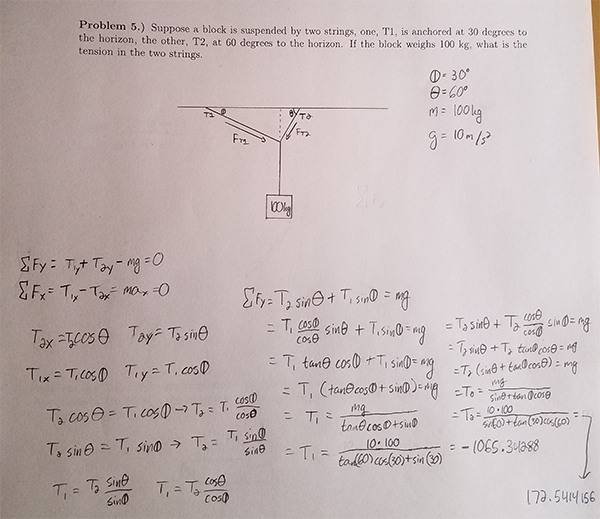# A Box is suspended by two strings, what is the tension?

1. The problem statement, all variables, and given/known data
Suppose a block is suspended by two strings, one, T1, is anchored at 30 degrees to the horizon, the other, T2, at 60 degrees to the horizon. If the block weighs 100 kg, what is the tension in the two strings?
Assume that:
The 30-degree angle is Phi.
The 60-degree angle is Theta.
M = 100 kg
g = 10 m/s^s

## Homework Equations

(I had to create them myself, so they will be shown in the attempt section.)

## The Attempt at a Solution

This image details my attempt. I am fairly certain that I got the correct answers, but I would really appreciate confirmation as well as an explanation for why certain things may be sin or cos. Most of the formulas are ones the professor just gave to us in class, and instead of expecting us to know how to create them, he just wants us to memorize them, which I struggle with unless I know the basis behind it.PeroK
Homework Helper
Gold Member
2020 Award
The key equation that you have is:

##T_1 = \frac{mg}{\tan(\theta) \cos(\phi) + \sin(\theta)}##

Which is very good!

In fact, if you then calculate a similar expression for ##T_2## you will see the symmetry of the solution.

kuruman